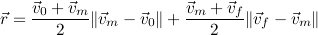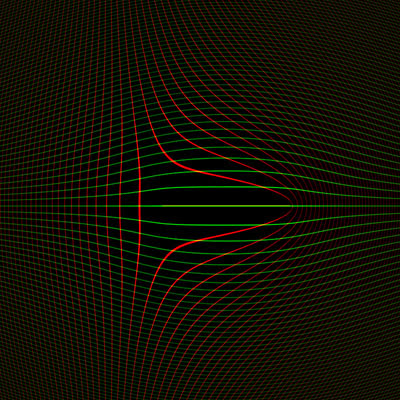## Monday, May 28, 2007

### Pathfinding in Space, part 3

Last week I ended with the vector equation I've been stuck trying to solve. vm is the unknown:The equation describes accelerating at a constant rate of 1 from a starting velocity v0 to an intermediate velocity vm, and then at the same rate of 1 (but possibly in a different direction) from vm to a final velocity vf. The desired displacement resulting from this trip is r.

Essentially we have position and velocity endpoint conditions, and a limit on how fast we can accelerate. My hypothesis is that the quickest path connecting the endpoints will be two parabolas (with the max acceleration on each) that fit the ends and match position and velocity where they meet.

I will probably put this problem on the back burner for a bit, but before doing so I thought I'd at least produce some graphs to get a better feel for it. I wrote a quick program that uses OpenGL to produce graphs of velocity space.

The program takes in a v0 and vf pair. Each point on the graph is a candidate vm; the program uses it to evaluate the right-hand side of the equation to give the r corresponding to that solution.

Lines of constant rx are drawn in red and lines of constant ry in green. If they were labeled, you could find a rough solution by locating the red line and green line that correspond to the desired r, and then locating an intersection between them. The position of the intersection is the value for vm that solves the equation.

Here is the simplest case, where the starting and ending velocities are both zero:(0, 0) to (0, 0)

The program dices the screen up into squares a few pixels on a side. For each square, it evaluates r at the four corners, then draws two textured quads additively over it. The first is drawn in red and uses rx as the Z coordinate. I've set OpenGL to map the Z value into a repeating one-dimensional texture which contains a few white pixels followed by a bunch of black ones. This creates the periodic lines. The texture is mipmapped to cut down on aliasing, so when the lines get really thin they fade out rather than dissolving into sparkles. The second quad is drawn in green, using ry as the Z coordinates for the quad corners.

Here's a slightly more complicated example, with starting velocity of zero:(0, 0) to (8, 0)

It looks like there is still a unique solution for every given displacement. The exception to this is on the line from (0, 0) to (8, 0) itself (drawn in yellow and faintly visible over the green); any point on there will give the same r.

Let's mix things up a bit more:(6, -2) to (6, 2)

Now there are closed loops of constant rx. There are some green and red lines that cross each other twice, indicating that for those values of r there are two solutions.

Here's a diagonal movement in velocity space:(5, 0) to (10, 5)

Now there are some red and green lines that cross each other three times! So there are at least some cases that have three solutions. I don't know if there are situations with four solutions. The minimum-time solution is the one closest to v0 and vf.

If nothing else, this would make a really wild screensaver, if you animated v0 and vf to bounce around the screen.

Amit said...

I probably don't understand all the constraints, but I think that in 2d or 3d space, the shortest path is still a straight line, just as in the 1d case. Instead of treating x and y separately, construct a new 1d “space” which is essentially the position u along the straight line from the start to the goal. Then use your 1d solution along this line, and convert back from u coordinates to x,y.

James McNeill said...

I'd love to get back to this project but I've been working on others for quite a while now. Hopefully I'm remembering right.

Even though the movement is in a 2D plane, because velocity is allowed to vary it is a part of the state space too. So the state space for the search has at least four dimensions.

Imagine that we want to get to the same position as where we currently are, but with a different velocity. A cost heuristic that considered only position (and not velocity too) would underestimate the cost for this maneuver, arbitrarily drastically depending on the desired change in velocity. This would result in many more nodes being searched. (Since the search space is four-dimensional it's easy to get an explosion of nodes searched anyway.)

Conversely, let's suppose that we want to get to a position (and velocity) which our current velocity will take us to on the next turn. A cost heuristic that considered only position might overestimate the cost to make this maneuver, which could result in the correct nodes not being searched at all.

Moe said...

14 years late, but did you ever finish this project ? I'm really intrested in seeing how you did it

James McNeill said...

Ha! No, I don't think I came up with a great solution. I am still trying to learn this stuff.

I think the two-parabola approach works but is not always optimal (although it's probably fairly good). The general approach for hunting for the values is to make some sort of objective function measuring how close we are to the solution, take a guess, take derivatives of the objective with respect to the variables, try moving some in a direction that should improve the objective, see if the objective improved, rinse and repeat. "Nonlinear programming" is the name of this game; essentially you're trying to move "downhill" from a starting point in search of a minimum in the objective function, at which the derivatives of the objective function will be zero in all variables. (Moving in any direction won't make the objective-function's value any better or worse.) There are tons of books about this, but hardly anybody writes the code to do it; they all rely on optimization libraries.

There's a thing called "linear tangent steering" in the older rocket literature that describes the actual time-optimal trajectory for this simple problem. Essentially what it's saying is that the solution will be as if there was a target moving through space with constant velocity, and you're aiming the rocket at that target throughout the maneuver. So in 2D you're solving for four unknowns (the target's starting position and velocity). However, you can scale that target trajectory in or out relative to the rocket's starting position (scaling the target's velocity as well), without changing how the rocket would steer. So there's an infinity of potential solutions, which means we have more degrees of freedom than we need. You could constrain it by saying that the target's starting position needs to be on the unit circle around the rocket, say. The search process for these coefficients could be done in a similar way, but figuring out what the derivatives are becomes much harder.

I remember seeing a paper from several decades back that was trying to do this. I think they integrated forward numerically using the guessed values for the target motion, remembering what the rocket orientation was at each time sub-step; then the derivatives (how changing the variables would change the motion) backward somehow to the start, to get derivatives to use to move the guess to the next step.

These days I think it's more common to use "direct transcription" to come up with an approximation to the trajectory. This is where you explode the optimization problem into a whole bunch of dimensions, by taking the discretization that you'd use to do the numerical integration, and making all of those intermediate states be part of the guess, subject to a whole bunch of constraints that the numerical integration actually matches the changes in states. Adding dimensions doesn't really help a ton for this simple linear-tangent steering problem, but it does let you take into account all kinds of other effects, like gravity or drag or the rocket's changing mass.